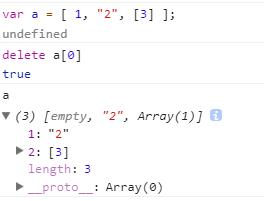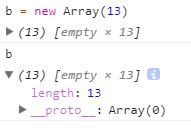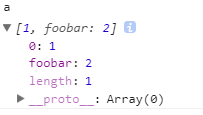js值----你所不知道的JavaScript系列（6）

1、数组

var a = [ 1, "2",  ];
a.length; // 3
a === 1; // true
a === 3; // true

var b = [ ];
b.length; // 0
b = 1;
b = "2";
b = [ 3 ];
b.length; // 3

var a = [ 1, "2",  ];
delete a;  // true
a.length;  // 3
a;  // [empty, "2", Array(1)]var a = [ ];
a = 1;
// 此处没有设置a单元
a = [ 3 ];
a; // undefined
a.length; // 3

var b = new Array(13);
b;  // [empty × 13]
b.length;  // 13var a = [ ];
a = 1;
a["foobar"] = 2;
a.length; // 1
a["foobar"]; // 2
a.foobar; // 2var c = [1,2,3,4,5];
c.length;  // 5
c;  // [1,2,3,4,5];

c.length = 3;
c;  // [1,2,3]

c.length = 6;
c;  // [1, 2, 3, empty × 3]

var a = [ ];
a["13"] = 13;
a.length; // 14

2、字符串

var a = "foo";
var b = ["f","o","o"];

a; // "o";
b; // "o";

a.length; // 3
b.length; // 3

a.indexOf( "o" ); // 1
b.indexOf( "o" ); // 1

var c = a.concat( "bar" ); // "foobar"
var d = b.concat( ["b","a","r"] ); // ["f","o","o","b","a","r"]

a === c; // false
b === d; // false

JavaScript 中字符串是不可变的，而数组是可变的。并且 a JavaScript 中并非总是合法语法，在老版本的 IE 中就不被允许（现在可以了）。 正确的方法应该是 a.charAt(1)。

var a = "foo";
a.length;  // 3

a.length = 2;

a;  // "foo"
a.length;  // 3

a.charAt(0);  // "f"

3、数字

JavaScript 只有一种数值类型： number（数字），包括“整数”和带小数的十进制数。此处“整数”之所以加引号是因为和其他语言不同， JavaScript 没有真正意义上的整数，这也是它一直以来为人诟病的地方。这种情况在将来或许会有所改观，但目前只有数字类型。  ----《你所不知道的JavaScript（中）》P15

JavaScript 中的“整数”就是没有小数的十进制数。所以 42.0 即等同于“整数” 42。

3.1 数字的语法

JavaScript 中的数字常量一般用十进制表示。例如：

var a = 42;
var b = 42.3;

var a = 0.42;
var b = .42;

var a = 42.0;
var b = 42.;  //42. 这?#20013;?#27861;没问题，只是不常见，但从代码的可?#21015;?#32771;虑，不建议这样写。

var a = 42.300;
var b = 42.0;
a; // 42.3
b; // 42

var a = 42.59;
a.toFixed( 0 ); // "43"
a.toFixed( 1 ); // "42.6"
a.toFixed( 2 ); // "42.59"
a.toFixed( 3 ); // "42.590"
a.toFixed( 4 ); // "42.5900"

上面的方法不仅适用于数字变量，也适用于数字常量。不过对于 . 运算符需要给予特别注意，因为它是一个有效的数字字符，会被优先识别为数字常量的一部分，然后才是对象属性访问运算符。

// 无效语法：
42.toFixed( 3 ); // SyntaxError
// 下面的语法?#21152;行В?/span>
(42).toFixed( 3 ); // "42.000"
0.42.toFixed( 3 ); // "0.420"
42..toFixed( 3 ); // "42.000
42 .toFixed(3); // "42.000"   注意.运算符前有空格

42.tofixed(3) 是无效语法，因为 . 被视为常量 42. 的一部分（如前所述），所以没有 . 性访问运算符来调用 tofixed 方法。

42..tofixed(3) 则没有问题，因为第一个 . 被视为 number 的一部分，第二个 . 是属性访问运算符。只是这样看着奇怪，实?#26159;?#20917;中也很少见。在基本类型值?#29616;?#25509;调用的方法并不多见，不过这并不代表不好不对。

3.2 较小的数值

0.1 + 0.2 === 0.3; // false

if (!Number.EPSILON) {
Number.EPSILON = Math.pow(2,-52);
}

function numbersCloseEnoughToEqual(n1,n2) {
return Math.abs( n1 - n2 ) < Number.EPSILON;
}
var a = 0.1 + 0.2;
var b = 0.3;
numbersCloseEnoughToEqual( a, b ); // true
numbersCloseEnoughToEqual( 0.0000001, 0.0000002 ); // false

3.3 整数的安全范围

Number.isSafeInteger( Number.MAX_SAFE_INTEGER ); // true
Number.isSafeInteger( Math.pow( 2, 53 ) ); // false
Number.isSafeInteger( Math.pow( 2, 53 ) - 1 ); // true

有时 JavaScript 程序需要处理一些比较大的数字，如数据库中的 64 ID 等。由于JavaScript 的数字类型无法精确呈现 64 位数值，所以必须将它们保存（转换）为字符串。

3.4 特殊数值

JavaScript 数据类型中有几个特殊的值需要开发人员特别注意和小心使用。

3.4.1　不是值的值

undefined 类型只有一个值，即 undefined。 null 类型也只有一个值，即 null。它们的名称既是类型也是值。

undefined 和 null 常被用来表示“空的”值或“不是值”的值。二者之间有一些细微的差别。例如：

• null 指空值（empty value）
• undefined 指没有值（missing value）

• undefined 指从未赋值
• null 指曾赋过值，但是目前没有值

null 是一个特殊关键字，不是标识符，我们不能将其当作变量来使用和赋值。然而undefined 却是一个标识符，可以被当作变量来使用和赋值。

3.4.2　undefined

function foo() {
undefined = 2; // 非常糟糕的做法！
}
foo();
function foo() {
"use strict";
undefined = 2; // TypeError!
}
foo();

function foo() {
"use strict";
var undefined = 2;
console.log( undefined ); // 2
}
foo();

void 运算符

undefined 是一个内置标识符（除非被重新定义，见前面的介绍），它的值为 undefined，通过 void 运算符即可得到该值。表达式 void ___ 没有返回值，因此返回结果是 undefined。 void 并不改变表达式的结果，只是让表达式不返回值：

var a = 42;
console.log( void a, a ); // undefined 42

3.5 特殊的数字

3.5.1 不是数字的数字

var a = 2 / "foo"; // NaN
typeof a === "number"; // true

NaN 是一个“警戒值”（sentinel value，有特殊用途的常规值），用于指出数字类型中的错误情况，即“执行数学运算没有成功，这是失败后返回的结果”。

NaN 是一个特殊值，它和自身不相等，是唯一一个非自反的值。即NaN==NaN为false，而 NaN != NaN true，很奇怪吧？ 那这样的话我们该如何比较和确定某个返回结果是否为NaN呢？

var a = 2 / "foo";
Number.isNaN(a);  // true

isNaN = function(n) {
return n !== n;
};

3.5.2 无穷数

var a = 1 / 0;

var a = 1 / 0; // Infinity
var b = -1 / 0; // -Infinity

3.6 零值

JavaScript 有一个常规的 0（也叫作 +0）和一个 -0。

-0 除了可以用作常量以外，也可以是某些数学运算的返回值。例如：

var a = 0 / -3; // -0
var b = 0 * -3; // -0

var a = 0 / -3;
a; // -0

a.toString(); // "0"
a + ""; // "0"
String( a ); // "0"

// JSON也如此
JSON.stringify( a ); // "0"

+"-0"; // -0
Number( "-0" ); // -0
JSON.parse( "-0" ); // -0

var a = 0;
var b = 0 / -3;
a == b; // true
-0 == 0; // true
a === b; // true
-0 === 0; // true
0 > -0; // false
a > b; // false

-0===0？那这样我们该如何判断是 0 还是 -0？可以?#20801;?#20197;下的方法：

function isNegZero(n) {
n = Number( n );
return (n === 0) && (1 / n === -Infinity);
}
isNegZero( -0 ); // true
isNegZero( 0 / -3 ); // true
isNegZero( 0 ); // false

3.7 特殊等式

var a = 2 / "foo";
var b = -3 * 0;
Object.is( a, NaN ); // true
Object.is( b, -0 ); // true
Object.is( b, 0 ); // false

Object.is = function(v1, v2) {
// 判断是否是-0
if (v1 === 0 && v2 === 0) {
return 1 / v1 === 1 / v2;
}
// 判断是否是NaN
if (v1 !== v1) {
return v2 !== v2;
}
// 其他情况
return v1 === v2;
};

posted @ 2018-08-01 11:01 李某龙 阅读(...) 评论(...) 编辑 收藏